# Wu Manber多模式匹配算法

AC自动机中，转移的最小单位是一个字符。也就是说，匹配后只能移动一个字符，复杂度是线性的$O(n)$。然而线性并非最快，Boyer-Moore算法在匹配后可以跳过多个字符，比线性还快。据说在实践中，利用Boyer-Moore优化的AC自动机总是更快。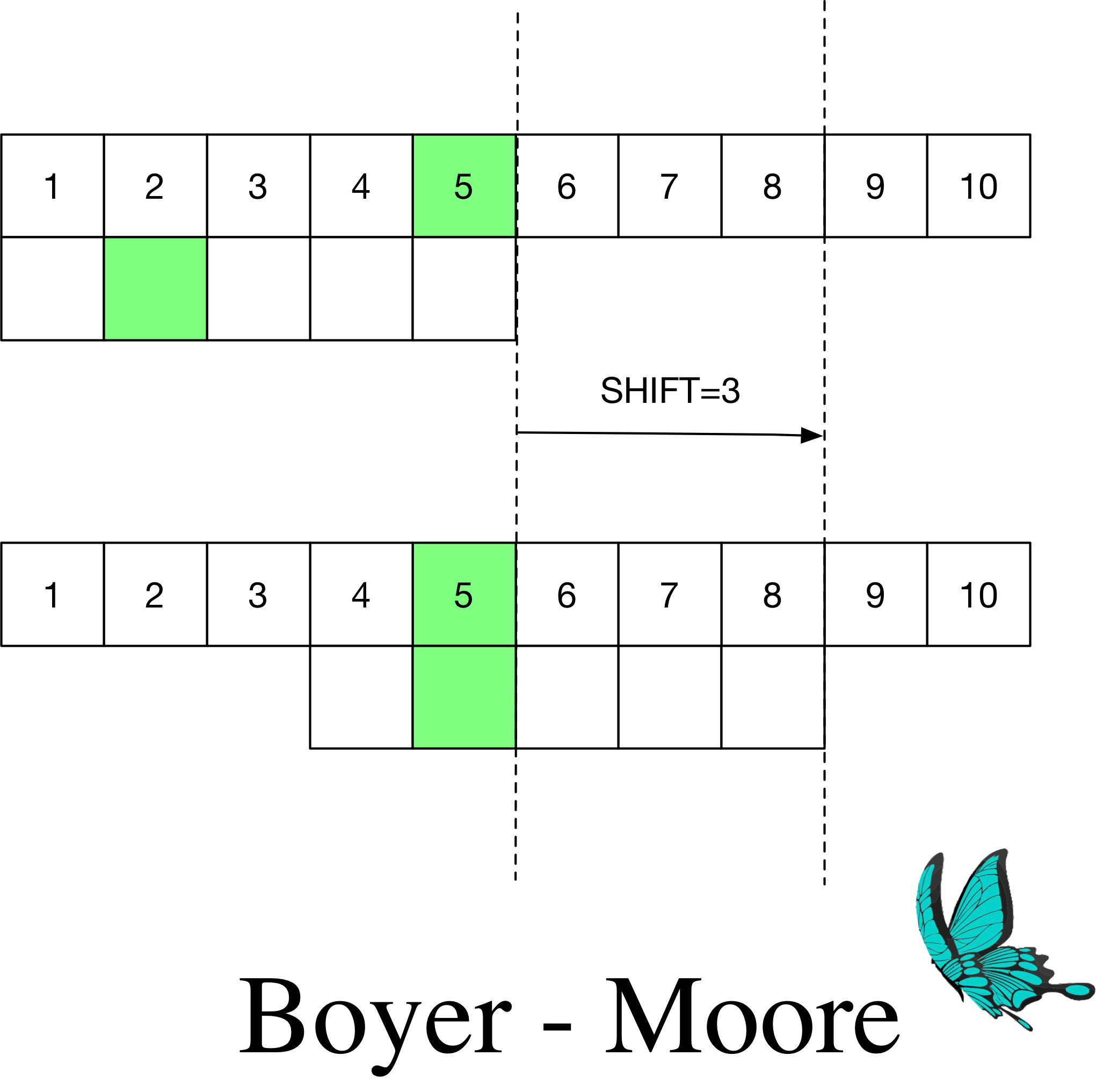Wu Manber利用了Boyer-Moore的思路，将该算法拓展到多模式匹配。

## 预处理

### SHIFT表的构造

SHIFT表还可以理解为，后缀作为子串在模式串中离尾部的最短距离（上图为$3$）。

1. 所有模式串都不含$X$
此时可以跳过$m-B+1$，将其存入SHIFT[i]中。

2. 存在包含$X$的模式串

### HASH表的构造

$SHIFT[h]=0$时，此时$HASH[h]$指向一个子链表的首部$p$，不断递增$p$直到$p+1$等于$HASH[h+1]$时即可得到子链表的尾部元素。

## 匹配

1. 计算母文本中当前长$B$的后缀$t_{m-B+1} \cdots t_m$的哈希值$h$。

2. 检查SHIFT[h]:如果$> 0$则跳过SHIFT[h]个字符并转到1；否则，转到3。

3. 计算当前位置往左$m$的长$B^\prime$的前缀的哈希值，记为text_prefix_hash

4. 检查$HASH[h]\leq p < HASH[h+1]$区间内的p是否有PREFIX(p)=text_prefix_hash，当两者相等时，进一步直接比较模式串与这段来自母文本的子串。当它们完全匹配的时候，就找到了一个模式串。无论找到与否，都将当前位置右移1个字符，并跳转1。

Oh et al. (2014)举了个例子：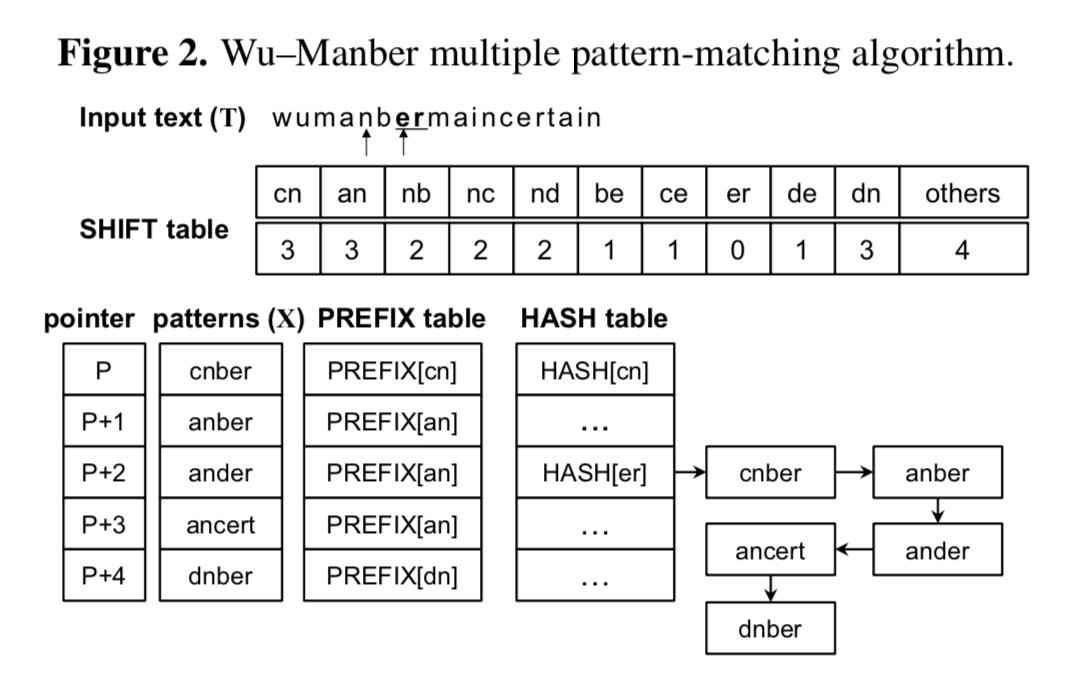## 复杂度

$P$个模式串，$M=mP$是所有模式串的总长度，最多$P=M/m$个子串对应同一个SHIFT值$i$（极端情况下所有模式串在位置$i$的子串都相等）。长$B$的子串最少有$M$个（极端情况下$B=1$，所有模式串长度都为$1$。这是我的理解，与论文给出的$2M$不同，我举出的反例如上所述）。所以随机挑一个子串，它的SHIFT值为某个特定值$i$的概率小于两者之比$1/m$。

## 变种

### ASHIFT表的构造

$m=5, B=1$，一共两个模式串。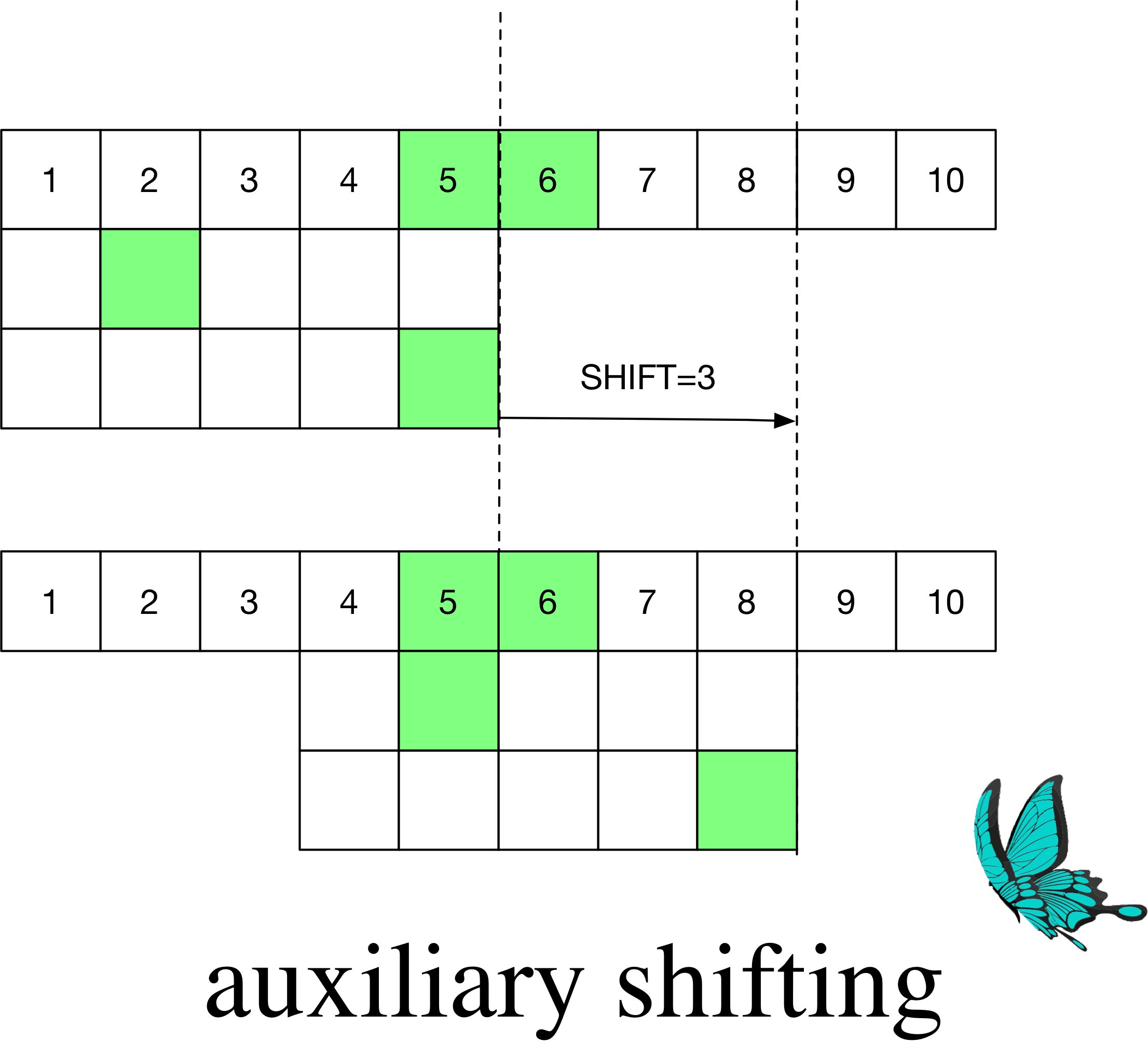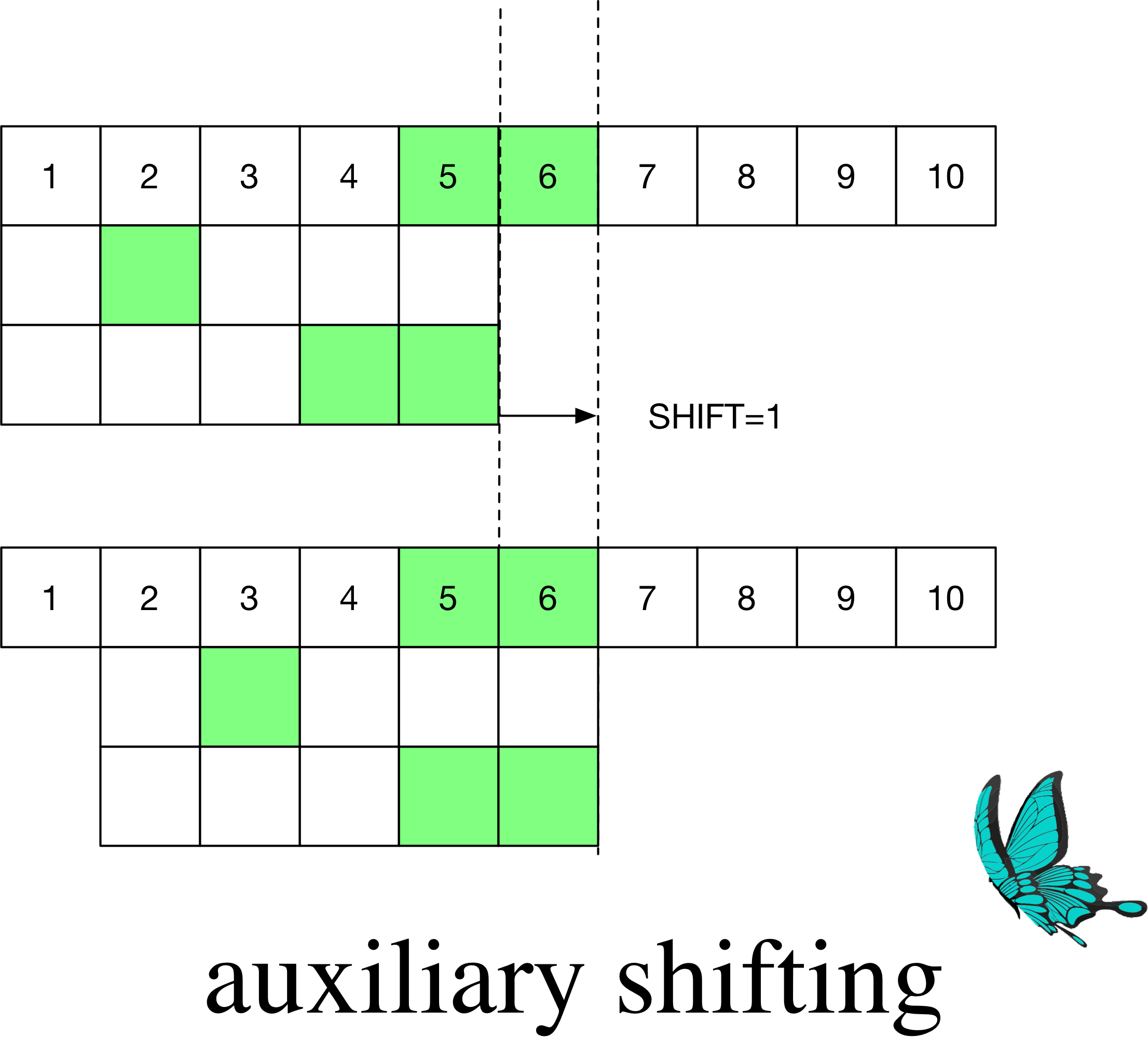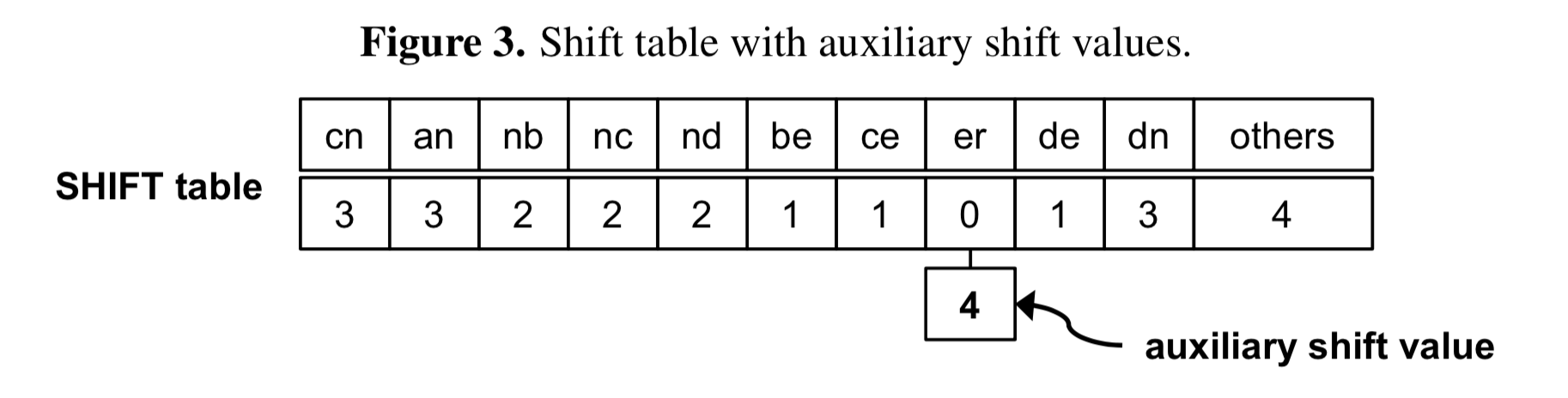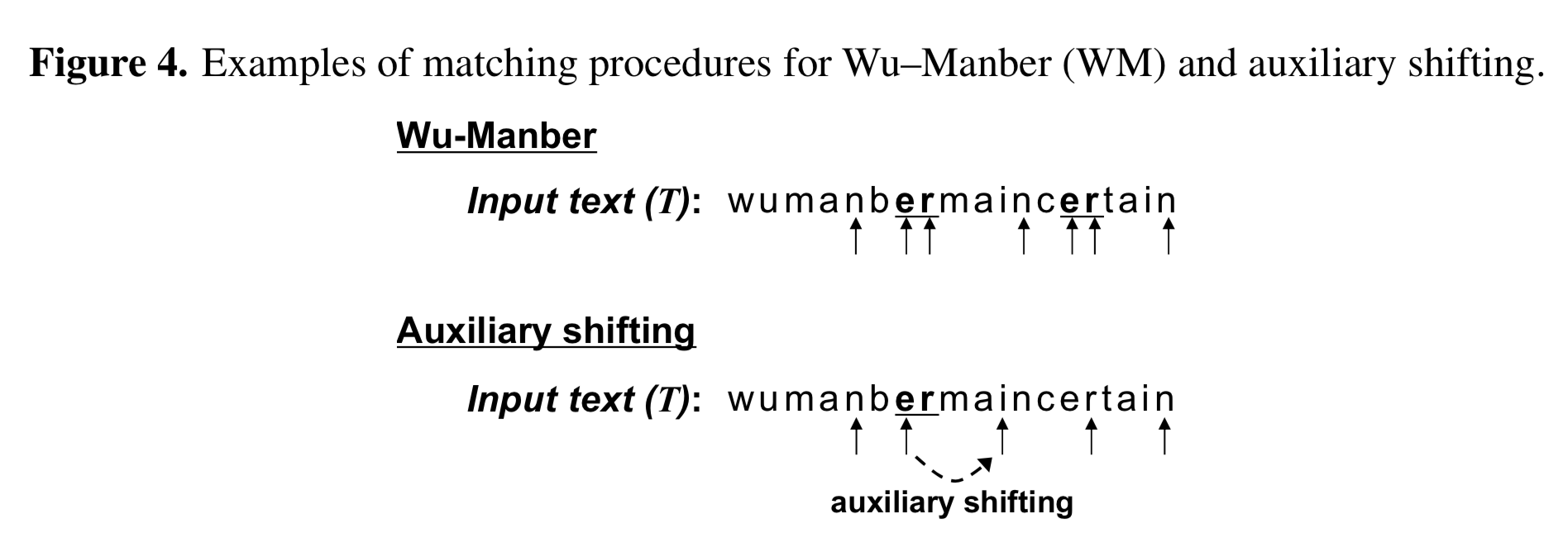## 总结

Wu Manber算法理论上复杂度为$O(BN/m)$($1 \leq B \leq m = min(strlen(M))$)，与AC自动机的$O(N)$相比，只有在特定条件下($B \ll m$)才能体现出优势。这个特定条件很苛刻，要求模式串不能太短。而在自然语言处理的场景下，经常有单字作为模式串的情况，此时Wu Manber无法跳过多个字符，没有优势。另外，汉语最常见的词语长度为$2$，也限制了该算法的使用。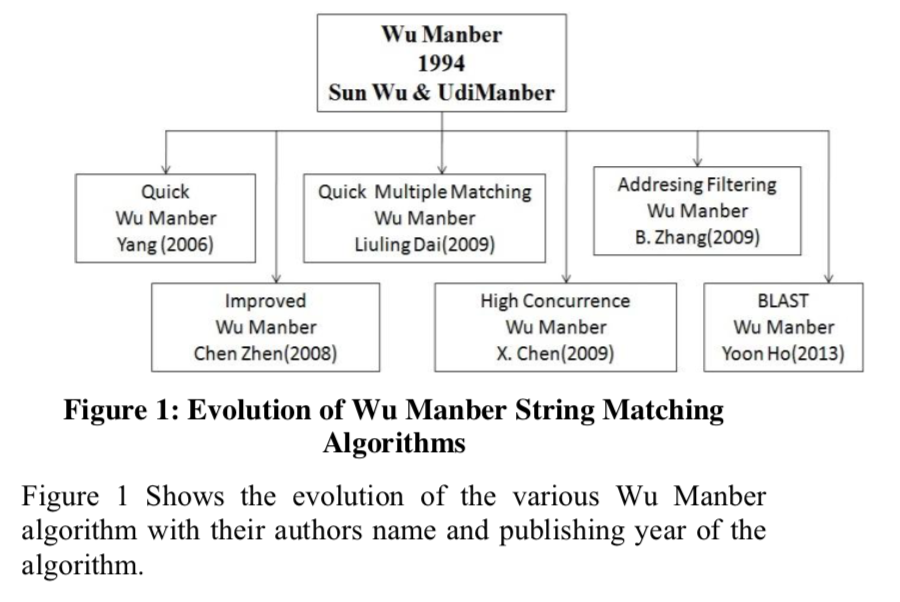## References

Wu S, Manber U. A fast algorithm for multi-pattern searching[J]. 1994.

D. Oh, D. Kim, and W. Ro, “A Malicious Pattern Detection Engine for Embedded Security Systems in the Internet of Things,” Sensors, vol. 14, no. 12, pp. 24188–24211, Dec. 2014.

V. Bhardwaj and V. Garg, “A Comparative Study of Wu Manber String Matching Algorithm and its Variations,” International Journal of Computer Applications, vol. 132, no. 17, pp. 34–38, Dec. 2015.

### 评论 2

1. #2

我的解决方法是将一个字的模式提取出来放到另外的组中使用单独的算法，其余的模式在一组中使用 这个算法。然后统一在外面包装一下，对使用者透明，效果不错。

6年前 (2018-03-02)回复
2. #1

大牛啊 持续关注

fly8wo6年前 (2018-02-04)回复